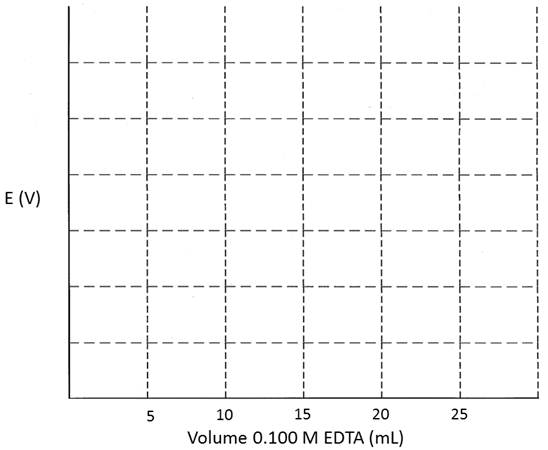# Electrochemical Titrations

1) 50.00 mL of a 0.034 M solution of Zn(SO4) buffered to pH = 10 is titrated with 0.1000 M EDTA.  The titration is monitored with a Zn(s) indicator electrode and a saturated Ag/AgCl reference electrode.  Calculate the measured potential at each point given below and then sketch the titration curve.

VEDTA = 2.5 mL:

VEDTA = 13 mL:

VEDTA = 17 mL:

VEDTA = 20 mL:2) 10.0 mL of 0.0500 M AgNO3 is titrated with 0.0250 M NaBr in the cell shown below:

$\textrm{SCE} \mid\mid \textrm{titration solution} \mid \textrm{Ag(s)}\nonumber$

Calculate the measured potential at each point given below and then sketch the titration curve.

VNaBr = 7.0 mL:

VNaBr = 19  mL:

VNaBr = 20 mL:

VNaBr = 25 mL:1.) Consider the titration of 50.0 mL of 0.100 M Fe2+ with 0.100 M Ce4+ in a solution of 1 F HClO4.  The indicator electrode is a Pt wire and the reference electrode is a saturated calomel electrode (SCE).

a.) There are two half-reactions that determine the potential at the indicator electrode.  Write these half-reactions and give their standard reduction potentials.

b.) Write the balanced, overall chemical equation for this reaction.

c.) What is the equivalent volume for this titration?

d.) Calculate the cell potential, Ecell, at a volume of 10.0 mL.

e.) Calculate the cell potential, Ecell, at a volume of 25.0 mL.

f.) What do you know about the concentrations of the four species in solution at the equivalence point?

g.) What is the cell potential, Ecell, at the equivalence point?

h.) What is the cell potential at a volume of 60.0 mL?

2.) Consider the titration of 50.0 mL of 0.0500 M Sn2+ with 0.100 M Tl3+ in 1 F HCl.  The indicator electrode is a Pt wire and the reference electrode is an Ag/AgCl electrode.

a.) There are two half-reactions that determine the potential at the indicator electrode.  Write these half-reactions and give their standard reduction potentials.

b.) Write the balanced, overall chemical equation for this reaction.

c.) What is the equivalent volume for this titration?

d.) Calculate the cell potential, Ecell, at a volume of 5.0 mL.

e.) Calculate the cell potential, Ecell, at a volume of 12.5 mL.

f.) What do you know about the concentrations of the four species in solution at the equivalence point?

g.) What is the cell potential, Ecell, at the equivalence point?

h.) What is the cell potential at a volume of 50.0 mL?